# Difference between revisions of "2070: Trig Identities"

 Trig Identities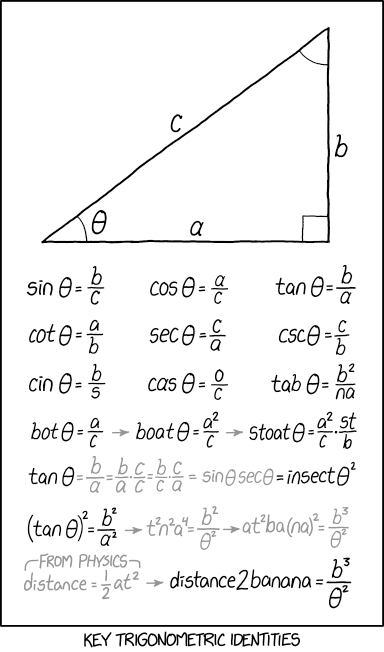Title text: ARCTANGENT THETA = ENCHANT AT TARGET

## ExplanationThis explanation may be incomplete or incorrect: Please only mention here why this explanation isn't complete. Do NOT delete this tag too soon.If you can address this issue, please edit the page! Thanks.

This comic shows several real and fictitious trigonometric identities. Most of the identities past the second line are "derived" by applying algebraic methods to the letters in the trig functions, which violates the rules of math, since the trig functions are operators and not variables.

The first line are well known trigonometric functions. The second line contains the lesser known reciprocals of the trigonometric functions in the first line. The following identities are made up and are increasing in absurdity. The comic reflects on the confusion one gets when working more intensely with these identities, since there are a lot of hidden dependencies between them.

The title-text is an anagram (which still has to be explained.)

## TranscriptThis transcript is incomplete. Please help editing it! Thanks.
[Inside a single frame comic a right-angled triangle is shown. The short edges are labeled "a" and "b" respectively and the long edge has a "c". All angles are marked, the right angle by a square and the both others by an arc. One arc is labeled by the Greek symbol theta.]
[Trigonometric functions on the marked angle theta in relation to "a", "b", and many more not depicted other variables are shown:]
[Caption below the frame:]
Key trigonometric identitiesadd a comment! ⋅add a topic (use sparingly)! ⋅refresh comments!

# Discussion

I am confused by the insect line. This seems to be true only if s=t. 141.101.96.209 19:03, 9 November 2018 (UTC)

I added a note regarding how similar it sounds to 'sinsec'. 172.68.51.154 01:47, 10 November 2018 (UTC)
That one and the cas aren't making any sense to me. GreatBigDot (talk) 20:02, 9 November 2018 (UTC)
Oh, the casinus is much important to... What was it? --Dgbrt (talk) 20:15, 9 November 2018 (UTC)
cas is realtively easy... it is cos(theta)=a/c -> cs(theta)=ao/c -> cas(theta)=o/c; when you realise that the top one isn't zero but o it clicks 141.101.96.209 23:35, 9 November 2018 (UTC)
You made the same error Randall did: you divided by 'o' on the left and multiplied on the right. I think the theme of the page is expanding significantly upon common math errors that were already humorous, like the common proof of 5=3 by dividing and multiplying by zero. The error here is in line with the theme of casual beginner errors. 172.68.51.154
You can see cin is derived from sin by swapping the positions of c and s. Likewise, Switching the a and o in cos(theta) = a/c gives cas(theta) = o/c i.e. no need for multiplicative consistency. The rule of treating things as a product of terms is implemented fully in the following lines. 162.158.91.83 11:23, 12 November 2018 (UTC)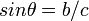$sin \theta = b/c$ leading to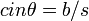$cin \theta = b/s$ is algebraically valid if you interpret sin as the product of s, i, n by multiplying both sides by c/s. It is not valid to just "swap" two letters in one equation that is part of a system of equations. You could do the same trick and get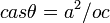$cas \theta = a^2/oc$ from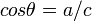$cos \theta = a/c$ or start with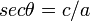$sec \theta = c/a$ and get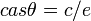$cas \theta = c/e$. Note for all equations except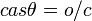$cas \theta = o/c$ and switching an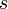$s$ to a$t$ to find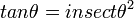$tan \theta = insect \theta^2$, the equations can be correctly derived by treating trig functions as product of single letter variables and algebraically manipulating them. Jimbob (talk) 16:59, 12 November 2018 (UTC)
I think insect is.. a bug.. ;) Smerriman (talk) 20:18, 9 November 2018 (UTC)

Is Enchant at target a magic:the gathering reference? AncientSwordRage (talk) 20:55, 9 November 2018 (UTC)

I think it is a Magic: The Gathering reference. Although it is phrased oddly. You'd think it would be "at target enchantment", rather than "target at enchantment". --Dryhamm (talk) 21:04, 9 November 2018 (UTC)
Likely, it refers to the bigbox retailer, Target. 172.68.58.233 (talk) (please sign your comments with ~~~~)
To me it sounds more like a reference to a nerdy video game, where a certain object worked like this, turning e.g. BEAM OF DARK ENERGY into a BAKED FERRY GNOME 108.162.246.11 21:53, 12 November 2018 (UTC)
Voila - s=t. -- Elliott (talk) (please sign your comments with ~~~~)
That was incredible! (assuming previous poster discovered the extrapolated proof in the description) 172.68.51.154 01:47, 10 November 2018 (UTC)
Combining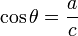$\cos\theta=\frac{a}{c}$ and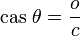$\mathrm{cas}\ \theta=\frac{o}{c}$ allows you to conclude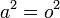$a^2 = o^2$, not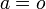$a=o$. 162.158.146.10 (talk) (please sign your comments with ~~~~)

Somebody added a comment on puns, e.g. that "cin sucks". More explanation is needed. It looks like some kind of a meta-joke. If you ask why, and start interpreting, you see that "b/c" == "because". It might be the answer to why the puns line should be removed, though. 172.68.51.154

For the Bot->Boat->Stoat line, this comes from the word game where you add/change letters to make a new word. Start with bot=a/c, multiply by a on both sides gets boat=a^2/c. Multiply by st on both sides and divide b on both sides gets Stoat=a^2/c*St/b. 162.158.78.166 (talk) (please sign your comments with ~~~~)

Uh... people... THE NAME GAME? Hello? https://en.wikipedia.org/wiki/The_Name_Game 162.158.79.107 (talk) (please sign your comments with ~~~~)

Checking through the math, just working from the real trig identities, without considering Randall's at-first-glance questionable identities like cas theta = o/c, basically everything that does not have a factor of d or 2 in it is equal to 1, and d is equal to 1/2, which then establishes the more questionable identities as tautological, 1=1. 162.158.142.100 04:09, 10 November 2018 (UTC)$\sec\theta = sect \eta$ 141.101.104.71 13:36, 10 November 2018 (UTC) AndreasH

Am I the only one who saw t²n²a⁴ as "tuna"? 172.68.58.233 14:17, 10 November 2018 (UTC)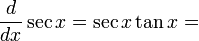$\frac{d}{dx}\sec x=\sec x\tan x=$ sex tanks. Probably not Douglas Hofstadter (talk) 21:36, 11 November 2018 (UTC)